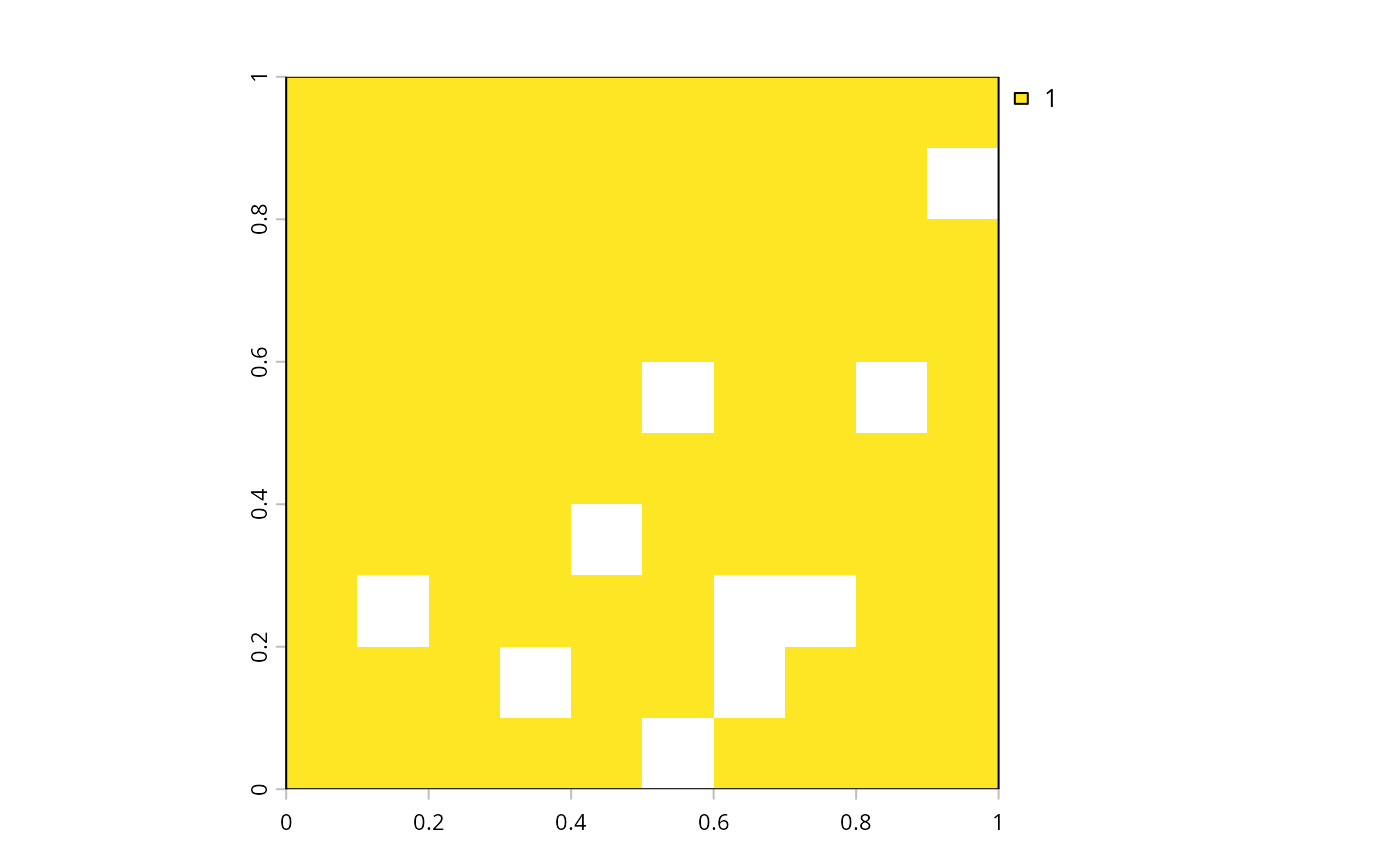Calculate the total abundance of each feature found in the planning units of a conservation planning problem.

feature_abundances(x, na.rm)

# S3 method for ConservationProblem
feature_abundances(x, na.rm = FALSE)

## Arguments

x

problem() (i.e., ConservationProblem) object.

na.rm

logical should planning units with NA cost data be excluded from the abundance calculations? The default argument is FALSE.

## Value

tibble::tibble() object containing the total amount ("absolute_abundance") and proportion ("relative_abundance") of the distribution of each feature in the planning units. Here, each row contains data that pertain to a specific feature in a specific management zone (if multiple zones are present). This object contains the following columns:

feature

character name of the feature.

zone

character name of the zone (not included when the argument to x contains only one management zone).

absolute_abundance

numeric amount of each feature in the planning units. If the problem contains multiple zones, then this column shows how well each feature is represented in a each zone.

relative_abundance

numeric proportion of the feature's distribution in the planning units. If the argument to na.rm is FALSE, then this column will only contain values equal to one. Otherwise, if the argument to na.rm is TRUE and planning units with NA cost data contain non-zero amounts of each feature, then this column will contain values between zero and one.

## Details

Planning units can have cost data with finite values (e.g., 0.1, 3, 100) and NA values. This functionality is provided so that locations which are not available for protected area acquisition can be included when calculating targets for conservation features (e.g., when targets are specified using add_relative_targets()). If the total amount of each feature in all the planning units is required---including the planning units with NA cost data---then the the na.rm argument should be set to FALSE. However, if the planning units with NA cost data should be excluded---for instance, to calculate the highest feasible targets for each feature---then the na.rm argument should be set to TRUE.

problem(), eval_feature_representation_summary().

## Examples

# load data
data(sim_pu_raster, sim_features)

# create a simple conservation planning dataset so we can see exactly
# how the feature abundances are calculated
pu <- data.frame(id = seq_len(10), cost = c(0.2, NA, runif(8)),
spp1 = runif(10), spp2 = c(rpois(9, 4), NA))

# create problem
p1 <- problem(pu, c("spp1", "spp2"), cost_column = "cost")

# calculate feature abundances; including planning units with NA costs
a1 <- feature_abundances(p1, na.rm = FALSE) # (default)
print(a1)
#> # A tibble: 2 × 3
#>   feature absolute_abundance relative_abundance
#>   <chr>                <dbl>              <dbl>
#> 1 spp1                  4.10                  1
#> 2 spp2                 31                     1

# calculate feature abundances; excluding planning units with NA costs
a2 <- feature_abundances(p1, na.rm = TRUE)
print(a2)
#> # A tibble: 2 × 3
#>   feature absolute_abundance relative_abundance
#>   <chr>                <dbl>              <dbl>
#> 1 spp1                  3.84              0.935
#> 2 spp2                 28                 0.903

# verify correctness of feature abundance calculations
all.equal(a1$absolute_abundance, c(sum(pu$spp1), sum(pu$spp2, na.rm = TRUE))) #>  TRUE all.equal(a1$relative_abundance,
c(sum(pu$spp1) / sum(pu$spp1),
sum(pu$spp2, na.rm = TRUE) / sum(pu$spp2, na.rm = TRUE)))
#>  TRUE

all.equal(a2$absolute_abundance, c(sum(pu$spp1[!is.na(pu$cost)]), sum(pu$spp2[!is.na(pu$cost)], na.rm = TRUE))) #>  TRUE all.equal(a2$relative_abundance,
c(sum(pu$spp1[!is.na(pu$cost)]) / sum(pu$spp1, na.rm = TRUE), sum(pu$spp2[!is.na(pu$cost)], na.rm = TRUE) / sum(pu$spp2,
na.rm = TRUE)))
#>  TRUE

# initialize conservation problem with raster data
p3 <- problem(sim_pu_raster, sim_features)

# calculate feature abundances; including planning units with NA costs
a3 <- feature_abundances(p3, na.rm = FALSE) # (default)
print(a3)
#> # A tibble: 5 × 3
#>   feature absolute_abundance relative_abundance
#>   <chr>                <dbl>              <dbl>
#> 1 layer.1               83.3                  1
#> 2 layer.2               31.2                  1
#> 3 layer.3               72.0                  1
#> 4 layer.4               42.7                  1
#> 5 layer.5               56.7                  1

# create problem using total amounts of features in all the planning units
# (including units with NA cost data)
p4 <- p3 %>%
add_relative_targets(a3$relative_abundance) %>% add_binary_decisions() %>% add_default_solver(verbose = FALSE) # attempt to solve the problem, but we will see that this problem is # infeasible because the targets cannot be met using only the planning units # with finite cost data # \dontrun{ s4 <- try(solve(p4)) #> Error in .local(a, b = b, ...) : #> no solution found (e.g., due to problem infeasibility or time limits) # } # calculate feature abundances; excluding planning units with NA costs a5 <- feature_abundances(p3, na.rm = TRUE) print(a5) #> # A tibble: 5 × 3 #> feature absolute_abundance relative_abundance #> <chr> <dbl> <dbl> #> 1 layer.1 74.5 0.895 #> 2 layer.2 28.1 0.900 #> 3 layer.3 64.9 0.902 #> 4 layer.4 38.2 0.895 #> 5 layer.5 50.7 0.893 # create problem using total amounts of features in the planning units with # finite cost data p5 <- p3 %>% add_min_set_objective() %>% add_relative_targets(a5$relative_abundance) %>%